# COVID-19 Data Visualization Python Project Class 12

## COVID-19 Data Visualization Python Project Class 12

# Python Project for Class 12 Informatics Practices (065), Python MySQL program containing bar graph, line chart, scatter chart

Note: If you found something wrong or unable to execute the program kindly mention in comment box provided below the article.

```#cbsepython.in
import pandas as pd
import numpy as np
import matplotlib.pyplot as plt

def Fun():
print(":)")
print("#1. For checking the data.")
print("#2. Reading complete file without index.")
print("===================")
print("Topic - Data Visualization")
print(" ")
print("#3. Line Chart")
print("    Press 1 to print the data for Confirmed cases as per Districts.")
print("    Press 2 to print the data for Recovered cases as per Districts.")
print("    Press 3 to print the data for Death cases as per Districts.")
print("    Press 4 to print the data for Active cases as per Districts.")
print("    Press 5 to print All data.")
print(" ")
print("#4. Bar Graph")
print("    Press 1 to print the data for Confirmed cases as per Districts.")
print("    Press 2 to print the data for Recovered cases as per Districts.")
print("    Press 3 to print the data for Death cases as per Districts.")
print("    Press 4 to print the data for Active cases as per Districts.")
print("    Press 5 to print the data in form of stack bar chart")
print("    Press 6 to print the data in form of multi bar chart")
print(" ")
print("#5. Scatter Chart")
print(" ")
print("#6. For Exit")
print("===============")

print("The Data")
print(df)

def No_Index():
print(df)

#FOR LINE CHART:)

def line_plot():
df['Districts'] = ['EKM','PTA','KTM','TSR','KKD','MPM','KLM','PKD','ALP','KNR','TVM','IDK','WYD','KGD']
District=df["Districts"]
Confirmed=df["Confirmed"]
Recovered=df["Recovered"]
Deaths=df["Deaths"]
Active=df["Active"]
plt.xlabel("Districts")

YC = int(input("Enter the number representing your preferred line chart from the above choices: "))

if YC == 1:
plt.ylabel("Confirmed Cases")
plt.title("Districts Wise Confirmed Cases")
plt.plot(District, Confirmed, color='b')
plt.show()
elif YC == 2:
plt.ylabel("Recovered Cases")
plt.title("Districts Wise Recovered Cases")
plt.plot(District, Recovered, color='g')
plt.show()
elif YC == 3:
plt.ylabel("Death Cases")
plt.title("Districts Wise Death Cases")
plt.plot(District, Deaths, color='r')
plt.show()
elif YC == 4:
plt.ylabel("Active Cases")
plt.title("Districts Wise Active Cases")
plt.plot(District, Active, color='c')
plt.show()
elif YC == 5:
plt.ylabel("Number of cases")
plt.plot(District, Confirmed, color='b', label = "Districts Wise Confirmed Cases")
plt.plot(District, Recovered, color='g', label = "Districts Wise Recovered Cases")
plt.plot(District, Deaths, color='r', label = "Districts Wise Death Cases")
plt.plot(District, Active, color='c', label = "Districts Wise Active Cases")
plt.legend()
plt.show()
else:
print("Enter valid input")

#FOR BAR GRAPH:)

def bar_plot():
df['Districts'] = ['EKM','PTA','KTM','TSR','KKD','MPM','KLM','PKD','ALP','KNR','TVM','IDK','WYD','KGD']
District = df["Districts"]
Confirmed = df["Confirmed"]
Recovered = df["Recovered"]
Deaths = df["Deaths"]
Active = df["Active"]
plt.xlabel("Districts")

YC = int(input("Enter the number representing your preferred bar graph from the above choices:"))

if YC == 1:
plt.ylabel("Confirmed Cases")
plt.title("Districts Wise Confirmed Cases")
plt.bar(District, Confirmed, color='b', width = 0.5)
plt.show()
elif YC == 2:
plt.ylabel("Recovered Cases")
plt.title("Districts Wise Recovered Cases")
plt.bar(District, Recovered, color='g', width = 0.5)
plt.show()
elif YC == 3:
plt.ylabel("Death Cases")
plt.title("Districts Wise Death Cases")
plt.bar(District, Deaths, color='r', width = 0.5)
plt.show()
elif YC == 4:
plt.ylabel("Active Cases")
plt.title("Districts Wise Active Cases")
plt.bar(District, Active, color='c', width = 0.5)
plt.show()
elif YC == 5:
plt.bar(District, Confirmed, color='b', width = 0.5, label = "Districts Wise Confirmed Cases")
plt.bar(District, Recovered, color='g', width = 0.5, label = "Districts Wise Recovered Cases")
plt.bar(District, Deaths, color='r', width = 0.5, label = "Districts Wise Death Cases")
plt.bar(District, Active, color='c',width = 0.5, label = "Districts Wise Active Cases")
plt.legend()
plt.show()
elif YC == 6:
D=np.arange(len(District))
width=0.25
plt.bar(D,Confirmed, width, color='b', label = "Districts Wise Confirmed Cases")
plt.bar(D+0.25, Recovered, width, color='g', label = "Districts Wise Recovered Cases")
plt.bar(D+0.50, Deaths, width, color='r', label = "Districts Wise Death Cases")
plt.bar(D+0.75, Active ,width, color='c', label = "Districts Wise Active Cases")
plt.legend()
plt.show()
else:
print("Enter valid input")

def scatter_chart():
df['Districts'] = ['EKM','PTA','KTM','TSR','KKD','MPM','KLM','PKD','ALP','KNR','TVM','IDK','WYD','KGD']
District = df["Districts"]
Confirmed = df["Confirmed"]
Recovered = df["Recovered"]
Deaths = df["Deaths"]
Active = df["Active"]

SC=plt.gca()
SC=plt.scatter(District, Confirmed, color="b", label="Districts Wise Confirmed Cases")
SC=plt.scatter(District, Recovered, color="g", label="Districts Wise Recovered Cases")
SC=plt.scatter(District, Deaths, color="r", label="Districts Wise Death Cases")
SC=plt.scatter(District, Active, color="c", label="Districts Wise Active Cases")

plt.xlabel("District")
plt.title("Complete Scatter Chart")
plt.legend()
plt.show()

Fun()
YC = int(input("Enter Your Choice: "))

while YC == 1 or 2 or 3 or 4 or 5 or 6:

if YC == 1:
break
elif YC == 2:
No_Index()
break
elif YC == 3:
line_plot()
break
elif YC == 4:
bar_plot()
break
elif YC == 5:
scatter_chart()
break
elif YC == 6:
print("Thank You for using...")
break
else:
print("Enter valid input")
break
```

Output:

```=== RESTART: C:\Users\ATC\AppData\Local\Programs\Python\Python38-32\COVID.PY
#1. For checking the data.
#2. Reading complete file without index.
===================
Topic - Data Visualization

#3. Line Chart
Press 1 to print the data for Confirmed cases as per Districts.
Press 2 to print the data for Recovered cases as per Districts.
Press 3 to print the data for Death cases as per Districts.
Press 4 to print the data for Active cases as per Districts.
Press 5 to print All data.

#4. Bar Graph
Press 1 to print the data for Confirmed cases as per Districts.
Press 2 to print the data for Recovered cases as per Districts.
Press 3 to print the data for Death cases as per Districts.
Press 4 to print the data for Active cases as per Districts.
Press 5 to print the data in form of stack bar chart
Press 6 to print the data in form of multi bar chart

#5. Scatter Chart

#6. For Exit
===============

```

### Screenshot :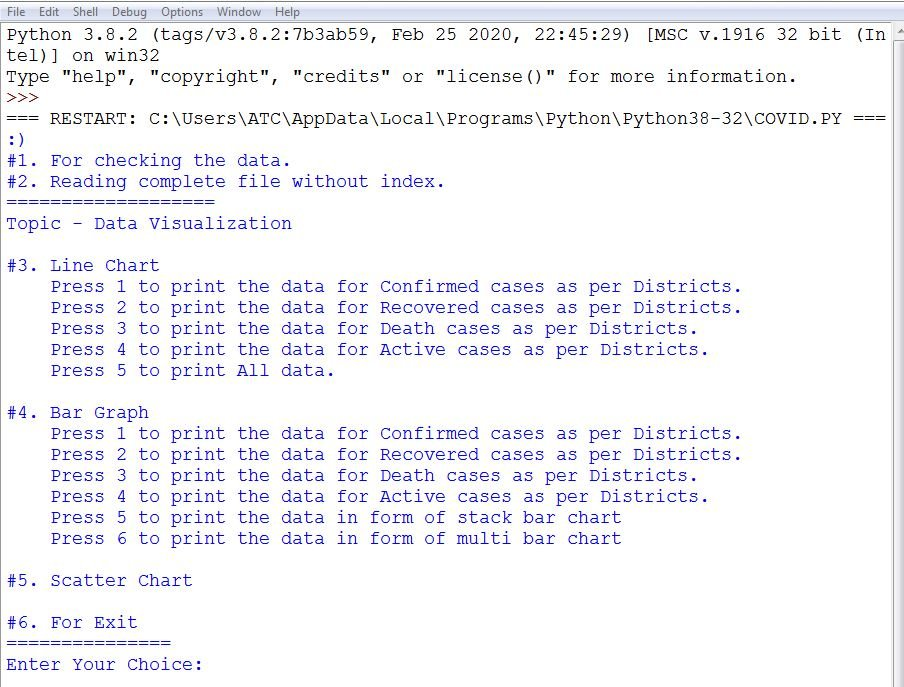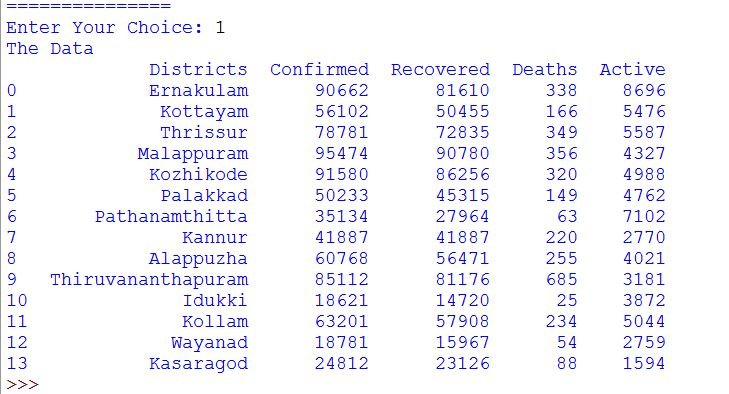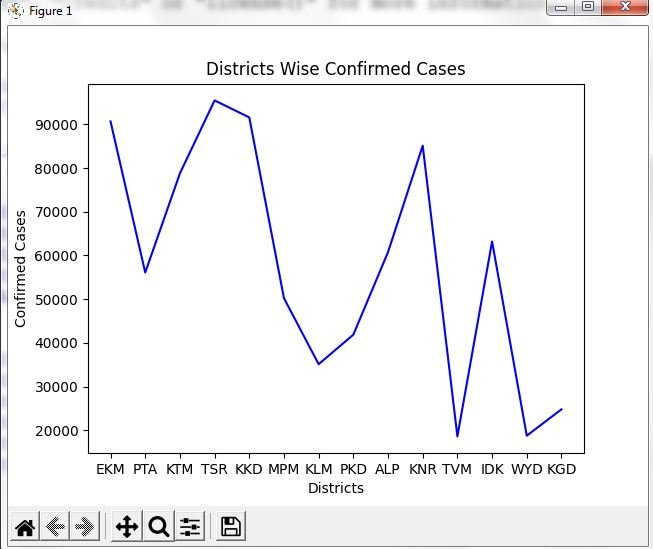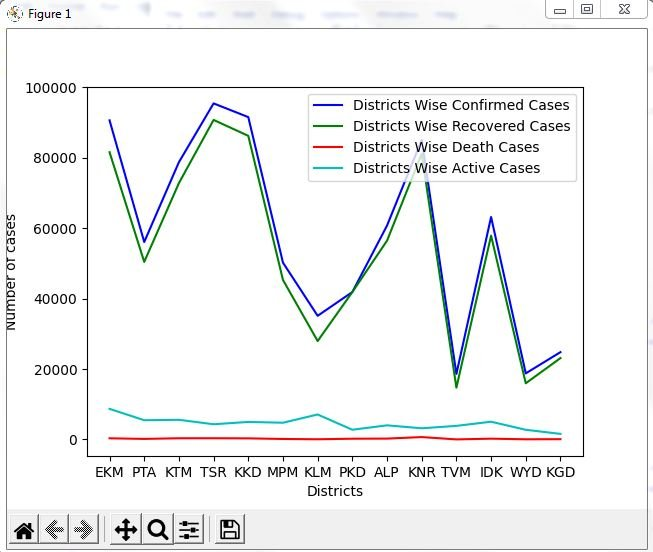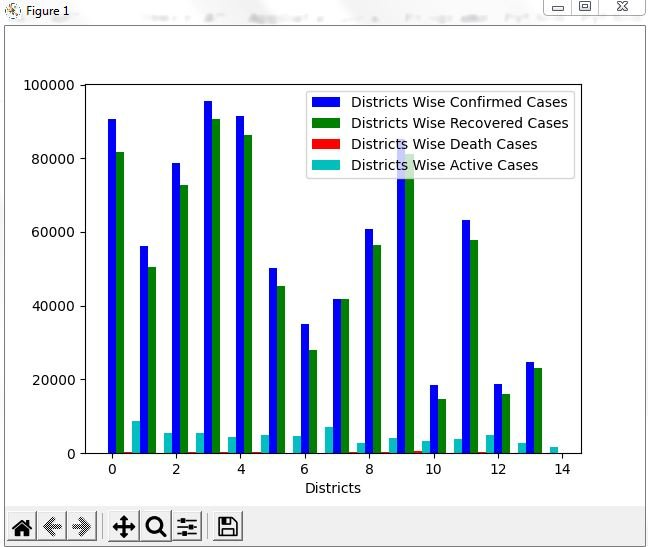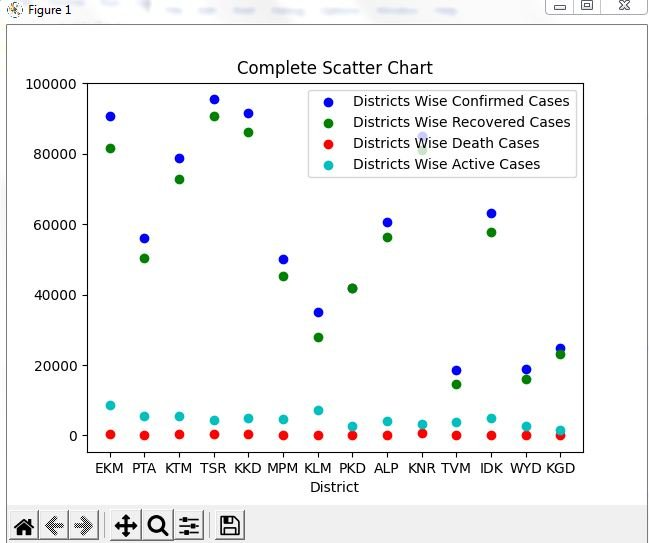#### By cbsepython

A complete solution for the students of class 9 to 12 having subject Information Technology (402), Computer Science (083). Explore our website for all the useful content as Topic wise notes, Solved QNA, MCQs, Projects and Quiz related to the latest syllabus.